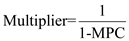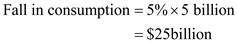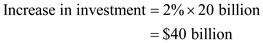## Quiz 29 : The Aggregate Expenditures ModelLooking for Economics Homework Help?# Quiz 29 : The Aggregate Expenditures Model

If the price level increases from P1 , which is \$80, to P3 , which is \$120, then we see that real GDP will decrease from Q1, which is \$300, to Q3 , which is \$100. Hence, real GDP will decrease by \$200. Since the aggregate expenditure schedule shifts downwards with an increase in price, we see that aggregate expenditure will shift downward when the price goes from \$80 to \$120. This shift will produce a decrease in real GDP from \$300 to \$200 (from Q1 to Q3 ). Remember that the multiplier is defined as:. Since we are given that the MPC is.8, we can simply substitute.8 for MPC in the expression for the multiplier, and we have:The Aggregate Expenditures Curve is plotted on a graph where the Y axis is aggregate expenditures and the X axis is real domestic output. The Aggregate Demand Curve is plotted on a graph where the Y axis is price level and the X axis is real domestic output. Note that the both X axis curves are the same, but the Y axis are different in the graph of the two curves. We see that an increase in price level will mean higher aggregate expenditures, which means the aggregate expenditure will actually shift up. The aggregate expenditures curve will shift up because price is not already on the Y-axis, but is something exogenous to the graph. Note that only variables that are not on the X-axis or Y-axis can shift a curve plotted on those X-axis and Y-axis. Now, an increase in the price level will not shift the aggregate demand curve because price is on the Y-axis of the graph for the aggregate demand curve. Since price is an endogenous variable to the graph (in other words, it's already represented on the Y-axis), a change in price can be shown by shifting along the aggregate demand curve. Another way to think about it is this; since the aggregate demand curve is plotted on a graph that has price and real domestic output as its Y-axis and X-axis, respectively, the aggregate demand curve should represent how a change in price level will affect real domestic output. This is exactly what the AD curve shows, and therefore a change in price will not shift the aggregate demand curve.

The real interest rate is the difference between the nominal interest rate and inflation rate. As per the problem, consumer spending increases by \$5 billion for every 1% increase in wealth of household. Also, the investment spending increases by \$20 billion for every 1% fall in the real interest rate. The multiplier is 4. Now, a wealth of household decreases by 5% due to a decrease in house value and real interest decreases by 2%. A 5 percent fall in household wealth will cause a \$25 billion fall in consumer spending. Numerically,Thus, the aggregate demand shifts to the left by \$25 billion. On the other hand, investment spending will increase by:Thus, investment spending will increase by \$40 billion because of the two percentage point decrease in real interest rate, since a one-percentage point decrease in investment will lead to a \$20 billion increase in investment spending. Thus, the aggregate demand shifts to the right by \$40 billion. The combined effect of the fall in consumption spending and an increase in investment spending will be:Hence, the aggregate demand curve will shift to the right by \$15 billion. The aggregate demand curve will shift right by \$15 billion in the initial stage. But due to the multiplier effect, the aggregate demand curve will shift further. Thus, the effect of the multiplier effect is as follow:With time, the multiplier effect of the economy will kick in. Since the economy's multiplier is 4, thus the aggregate demand curve will eventually shift rightward by \$60 billion.

There is no answer for this question

There is no answer for this question